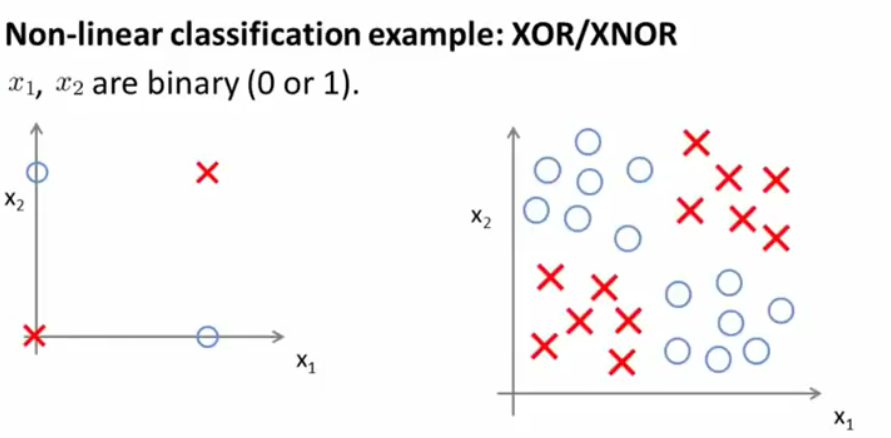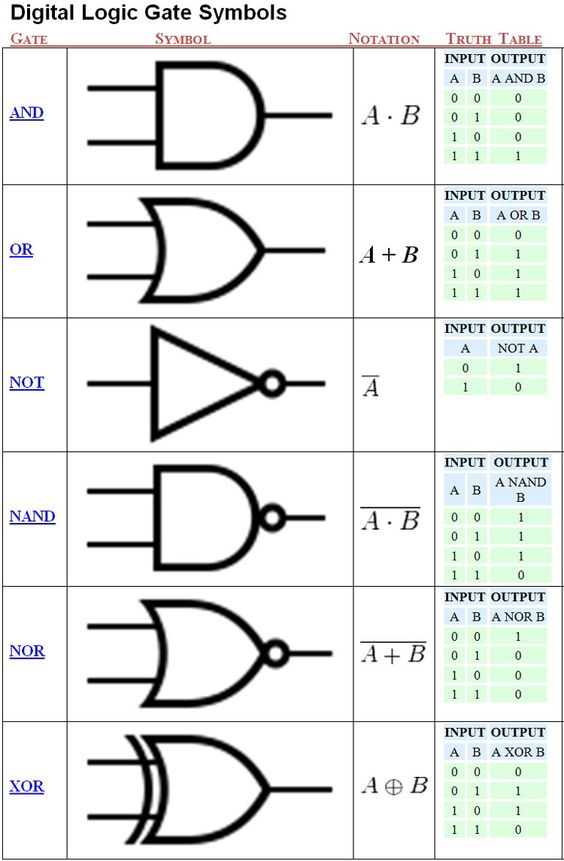### EXAMPLE OF NON-LINEAR CLASSIFICATION:

From Andrew Ng ML course:Simplifying the classification problem on the right as a binary $$0/1$$ $$x_1$$ and $$x_2$$ prediction we have the equivalent of an XOR gate:In fact, if the circles are zeros, the model is more of an XNOR function: $$y = x_1 \text{XNOR} x_2 = \text{NOT} (x_1 \text{XOR} x_2)$$.